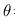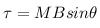Courses

# Test: Magnetism And Matter (CBSE Level With Solution)

## 30 Questions MCQ Test Physics Class 12 | Test: Magnetism And Matter (CBSE Level With Solution)

Description
This mock test of Test: Magnetism And Matter (CBSE Level With Solution) for Class 12 helps you for every Class 12 entrance exam. This contains 30 Multiple Choice Questions for Class 12 Test: Magnetism And Matter (CBSE Level With Solution) (mcq) to study with solutions a complete question bank. The solved questions answers in this Test: Magnetism And Matter (CBSE Level With Solution) quiz give you a good mix of easy questions and tough questions. Class 12 students definitely take this Test: Magnetism And Matter (CBSE Level With Solution) exercise for a better result in the exam. You can find other Test: Magnetism And Matter (CBSE Level With Solution) extra questions, long questions & short questions for Class 12 on EduRev as well by searching above.
QUESTION: 1

### Which of the following statements about earth's magnetism is correct

Solution:

According to recent researches the magnetic field of earth is considered due to a large bar magnet situated in earth's core.
It is considered that the north pole of this large magnet is situated at the geographical south of earth and vice versa and as the magnetic field due to a bar magnet is from north pole to south pole of the maget thus the earth's magnetic field is considered from geographical south to geographical north which are respectively north and south poles of the bar magnet.

QUESTION: 2

Solution:
QUESTION: 3

### A toroid wound with 60.0 turns/m of wire carries a current of 5.00 A. The torus is iron, which has a magnetic permeability of μm=5000μ0 under the given conditions. H and B inside the iron are

Solution:
QUESTION: 4

A bar magnet of magnetic moment 1.5 J/T lies aligned with the direction of a uniform magnetic field of 0.22 T. What is the amount of work required by an external torque to turn the magnet so as to align its magnetic moment opposite to the field direction?

Solution:
QUESTION: 5

In the magnetic meridian of a certain place, the horizontal component of the earth’s magnetic field is 0.26G and the dip angle is 600. What is the magnetic field of the earth at this location

Solution:
QUESTION: 6

Which of the following statements about bar magnets is correct ?

Solution:

Explanation:As magnetic monopole does not exist. If we split the bar magnet into two pieces  each part will have its own north and south pole.

QUESTION: 7

Magnetic material differences are explained by

Solution:
QUESTION: 8

A cube-shaped permanent magnet is made of a ferromagnetic material with a magnetization M of about The side length is 2 cm. Magnetic dipole moment of the magnet is.

Solution:
QUESTION: 9

A bar magnet of magnetic moment 1.5 J/T lies perpendicular to the direction of a uniform magnetic field of 0.22 T. What is the torque acting on it?

Solution:
QUESTION: 10

A short bar magnet placed in a horizontal plane has its axis aligned along the magnetic north-south direction. Null points are found on the axis of the magnet at 14 cm from the centre of the magnet. The earth’s magnetic field at the place is 0.36 G and the angle of dip is zero. What is the total magnetic field on the normal bisector of the magnet at the same distance as the null–point (i.e., 14 cm) from the centre of the magnet? (At null points, field due to a magnet is equal and opposite to the horizontal component of earth’s magnetic field.)

Solution:
QUESTION: 11

When iron filings are sprinkled on a sheet of glass placed over a short bar magnet then,

Solution:

Explanation:North pole act as positive end and South pole act as negative end. The iron fillings in the presence of magnetic field  gets magnetized and form a tiny magnet which then gets  attracted to the poles of bar magnet. North pole of bar magnet attracts south pole of tiny magnet and vice-versa. So all the iron fillings are arranged as the magnetic field lines of bar magnet.

QUESTION: 12

Diamagnetic substances are

Solution:
QUESTION: 13

A cube-shaped permanent magnet is made of a ferromagnetic material with a magnetization M of about The side length is 2 cm. Magnetic field due to the magnet at a point 10 cm from the magnet along its axis is

Solution:
QUESTION: 14

A bar magnet of magnetic moment 1.5 J/T lies parallel to the direction of a uniform magnetic field of 0.22 T. What is the torque acting on it?

Solution:

Solution :- torque when=180°= 1.5 × 0.22 × sin 180°

= 0.33 × 0 = 0 Nm

QUESTION: 15

A short bar magnet of magnetic moment 5.25×10−2JT−1 is placed with its axis perpendicular to the earth’s field direction. At what distance from the centre of the magnet, the resultant field is inclined at 45 with earth’s field on its normal bisector . Magnitude of the earth’s field at the place is given to be 0.42 G. Ignore the length of the magnet in comparison to the distances involved.

Solution:
QUESTION: 16

Which of the following statements about magnetic field lines true?

Solution:
QUESTION: 17

For diamagnetic materials

Solution:
QUESTION: 18

permeability of a paramagnetic material is expected to decrease with increasing temperature because

Solution:
QUESTION: 19

A magnetic needle free to rotate in a vertical plane parallel to the magnetic meridian has its north tip pointing down at 220 with the horizontal. The horizontal component of the earth’s magnetic field at the place is known to be 0.35 G. Determine the magnitude of the earth’s magnetic field at the place.

Solution:
QUESTION: 20

A Rowland ring of mean radius 15 cm has 3500 turns of wire wound on a ferromagnetic core of relative permeability 800. What is the magnetic field B in the core for a magnetising current of  12 A ?

Solution:
QUESTION: 21

In which case of comparing solenoid and bar magnet there is no exact similarity?

Solution:
QUESTION: 22

For paramagnetic materials

Solution:
QUESTION: 23

If a magnet is suspended over a container of liquid air, it attracts droplets to its poles. The droplets contain only liquid oxygen and no nitrogen because

Solution:
QUESTION: 24

A short bar magnet has a magnetic moment of 0.48 J/T .Magnetic field produced by the magnet at a distance of 10 cm from the centre of the magnet on the axis has a direction and magnitude of .

Solution:
QUESTION: 25

A compass needle free to turn in a horizontal plane is placed at the centre of circular coil of 30 turns and radius 12 cm. The coil is in a vertical plane making an angle of 450 with the magnetic meridian. When the current in the coil is 0.35 A, the needle points west to east. Determine the horizontal component of the earth’s magnetic field at the location

Solution:
QUESTION: 26

Far axial magnetic field of a solenoid as well as bar magnet vary

Solution:
QUESTION: 27

In Ferromagnetic materials

Solution:
QUESTION: 28

which of the following features of atomic structure determine whether an element is diamagnetic or paramagnetic

Solution:

Spin and Orbital angular momentum arise due to the presence of unpaired electrons. If there are unpaired electrons, these momenta will be there otherwise not.

QUESTION: 29

A toroidal solenoid with 500 turns is wound on a ring with a mean radius of 2.90 cm. Find the current in the winding that is required to set up a magnetic field of 0.350 T in the ring if the ring is made of annealed iron Km=1400

Solution:
QUESTION: 30

Correct unit of Bohr magneton is

Solution: